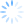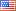## Trie树

Trie树也称字典树，因为其效率很高，所以在在字符串查找、前缀匹配等中应用很广泛，其高效率是以空间为代价的。

```#define MAX 26

typedef struct TrieNode               //Trie结点声明
{
bool isStr;                      //标记该结点处是否构成单词
struct TrieNode *next[MAX];      //儿子分支
}Trie;```Trie树的根结点不包含任何信息，第一个字符串为"abc"，第一个字母为'a'，因此根结点中数组next下标为'a'-97的值不为NULL，其他同理，构建的Trie树如图所示，红色结点表示在该处可以构成一个单词。很显然，如果要查找单词"abc"是否存在，查找长度则为O(len)，len为要查找的字符串的长度。而若采用一般的逐个匹配查找，则查找长度为O(len*n)，n为字符串的个数。显然基于Trie树的查找效率要高很多。

1.插入

1)取str[i]，判断p->next[str[i]-97]是否为空，若为空，则建立结点temp，并将p->next[str[i]-97]指向temp，然后p指向temp；

2)i++，继续取str[i]，循环1)中的操作，直到遇到结束符'\0'，此时将当前结点p中的isStr置为true。

2.查找

1)取str[i]，判断判断p->next[str[i]-97]是否为空，若为空，则返回false；若不为空，则p=p->next[str[i]-97]，继续取字符。

2)重复1)中的操作直到遇到结束符'\0',若当前结点p不为空并且isStr为true，则返回true，否则返回false。

3.删除

```/*Trie树(字典树) 2011.10.10*/
#include <iostream>
#include<cstdlib>
#define MAX 26
using namespace std;
typedef struct TrieNode //Trie结点声明
{
bool isStr; //标记该结点处是否构成单词
struct TrieNode *next[MAX]; //儿子分支
}Trie;
void insert(Trie *root,const char *s) //将单词s插入到字典树中
{
if(root==NULL||*s=='\0')
return;
int i;
Trie *p=root;
while(*s!='\0')
{
if(p->next[*s-'a']==NULL) //如果不存在，则建立结点
{
Trie *temp=(Trie *)malloc(sizeof(Trie));
for(i=0;i<MAX;i++)
{
temp->next[i]=NULL;
}
temp->isStr=false;
p->next[*s-'a']=temp;
p=p->next[*s-'a'];
}
else
{
p=p->next[*s-'a'];
}
s++;
}
p->isStr=true; //单词结束的地方标记此处可以构成一个单词
}
int search(Trie *root,const char *s) //查找某个单词是否已经存在
{
Trie *p=root;
while(p!=NULL&&*s!='\0')
{
p=p->next[*s-'a'];
s++;
}
return (p!=NULL&&p->isStr==true); //在单词结束处的标记为true时，单词才存在
}
void del(Trie *root) //释放整个字典树占的堆区空间
{
int i;
for(i=0;i<MAX;i++)
{
if(root->next[i]!=NULL)
{
del(root->next[i]);
}
}
free(root);
}
int main(int argc, char *argv[])
{
int i;
int n,m; //n为建立Trie树输入的单词数，m为要查找的单词数
char s;
Trie *root= (Trie *)malloc(sizeof(Trie));
for(i=0;i<MAX;i++)
{
root->next[i]=NULL;
}
root->isStr=false;
scanf("%d",&n);
getchar();
for(i=0;i<n;i++) //先建立字典树
{
scanf("%s",s);
insert(root,s);
}
while(scanf("%d",&m)!=EOF)
{
for(i=0;i<m;i++) //查找
{
scanf("%s",s);
if(search(root,s)==1)
printf("YES\n");
else
printf("NO\n");
}
printf("\n");
}
del(root); //释放空间很重要
return 0;
}```

Posted in: 技术文章

Tags: , , ,### 添加评论• 评论
• 在线预览